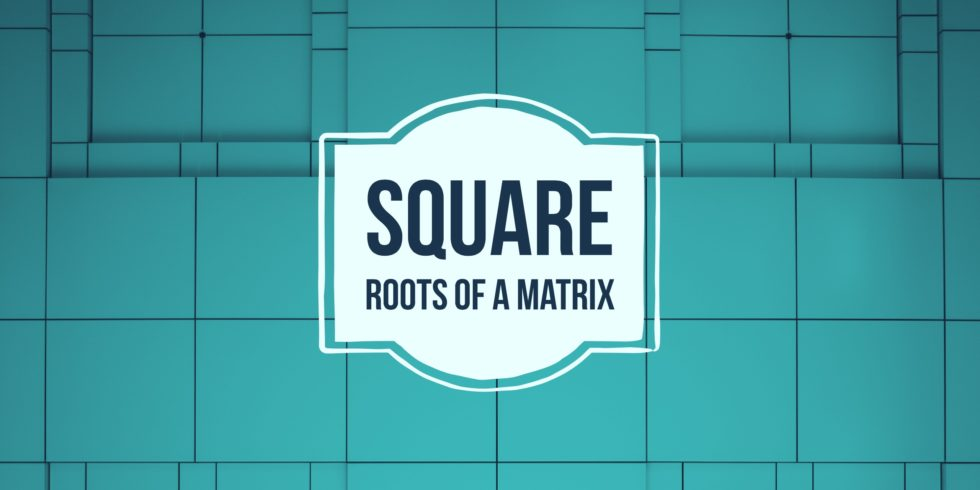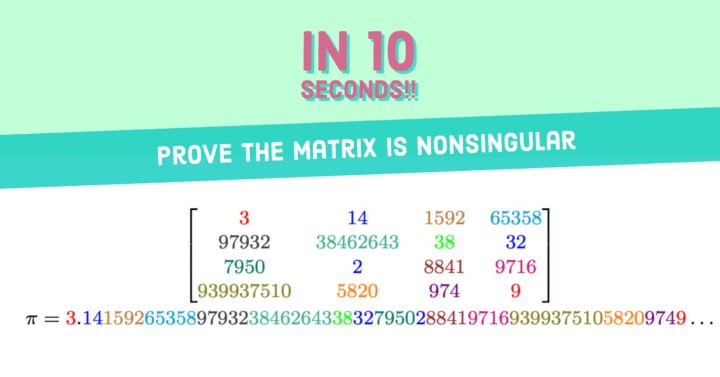# No/Infinitely Many Square Roots of 2 by 2 Matrices## Problem 512

(a) Prove that the matrix $A=\begin{bmatrix} 0 & 1\\ 0& 0 \end{bmatrix}$ does not have a square root.
Namely, show that there is no complex matrix $B$ such that $B^2=A$.

(b) Prove that the $2\times 2$ identity matrix $I$ has infinitely many distinct square root matrices.Add to solve later

## Proof.

### (a) The matrix $A$ does not have a square root.

Let $B=\begin{bmatrix} a & b\\ c& d \end{bmatrix}$ be the matrix such that $B^2=A$.
Since
\begin{align*}
B^2=\begin{bmatrix}
a & b\\
c& d
\end{bmatrix}\begin{bmatrix}
a & b\\
c& d
\end{bmatrix}
=\begin{bmatrix}
a^2+bc & ab+bd\\
ca+dc & cb+d^2
\end{bmatrix}
=\begin{bmatrix}
a^2+bc & (a+d)b\\
(a+d)c & cb+d^2
\end{bmatrix},
\end{align*}
it follows from $B^2=A$ that
$\begin{bmatrix} a^2+bc & (a+d)b\\ (a+d)c & cb+d^2 \end{bmatrix}=\begin{bmatrix} 0 & 1\\ 0& 0 \end{bmatrix}.$

Comparing entries we obtain four equations
\begin{align*}
a^2+bc&=0 \tag{1}\\
(a+d)b&=1\tag{2}\\
(a+d)c&=0\tag{3}\\
cb+d^2&=0.\tag{4}
\end{align*}

Equation (3) gives $a+d=0$ or $c=0$.
If $a+d=0$, then equation (2) becomes $0=1$. This is impossible and thus $c=0$.
Since $c=0$, equations (1) and (4) yield that $a=d=0$.
However, inserting these into (2) gives $0=1$.
Hence there is no solution satisfying these equations.

Therefore, there is no square root of the matrix $A$.

### (b) The identity matrix has infinitely many square roots

Let
$B=\begin{bmatrix} 1 & r\\ 0& -1 \end{bmatrix},$ where $r$ be an arbitrary real numbers.

Then we compute directly and obtain
\begin{align*}
B^2=\begin{bmatrix}
1 & r\\
0& -1
\end{bmatrix}\begin{bmatrix}
1 & r\\
0& -1
\end{bmatrix}=\begin{bmatrix}
1 & 0\\
0& 1
\end{bmatrix}=I.
\end{align*}

Hence $B$ is a square root of the identity matrix $I$.
Since $r$ is an arbitrary real numbers, there are infinitely many square roots of $I$.

## Related Question.

Problem.
Prove that a positive definite matrix has a unique positive definite square root.

For a solution of this problem, see the post
A Positive Definite Matrix Has a Unique Positive Definite Square RootAdd to solve later

### More from my site

#### You may also like...

This site uses Akismet to reduce spam. Learn how your comment data is processed.

###### More in Linear Algebra##### How to Prove a Matrix is Nonsingular in 10 Seconds

Using the numbers appearing in $\pi=3.1415926535897932384626433832795028841971693993751058209749\dots$ we construct the matrix \[A=\begin{bmatrix} 3 & 14 &1592& 65358\\ 97932& 38462643& 38& 32\\...

Close